March 12, 2017

# Kernel Compositions

KernelCompositions operate enriching the computation of another kernel. Details on these popular kernels can be found in (Shawe-Taylor and Cristianini, 2004).

### Polynomial Kernel

Java class: PolynomialKernel

Source code: PolynomialKernel.java

Maven Project: kelp-core

JSON type: poly

Description: it implicitly works in a features space where all the polynomials of the original features are available. As an example, a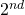degree polynomial kernel applied over a linear kernel on vector representations will automatically consider pairs of features in its similarity evaluation. Given a base kernel K,  the PolynomialKernel applies the following formula: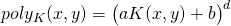where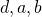are kernel parameters. Common values are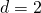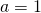and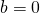.

Parameters:

• baseKernel: the base kernel whose output is enriched by this kernel
• a: the coefficient a in the formula above
• b: the coefficient b in the formula above
• degree: the degree d of the formula above

Java class: RbfKernel

Source code: RbfKernel.java

Maven Project: kelp-core

JSON type: rbf

Description: the Radial Basis Function (RBF) Kernel, a.k.a. Gaussian Kernel, enriches another kernel according to the following formula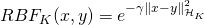where: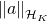is the norm of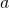in the kernel space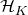generated by a base kernel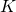.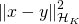can be computed as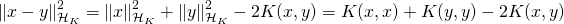. This allows to apply the RBF operation to any kernel base kernel K. It depends on a width  parameter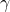which regulates how fast the RbfKernel similarity decays w.r.t. the distance of the input objects in. It can be proven that the Gaussian Kernel produces an infinite dimensional RKHS.

Parameters:

• baseKernel: the base kernel whose output is enriched by this kernel
• gamma: the gamma parameter in the formula above

### Normalization Kernel

Java class: NormalizationKernel

Source code: NormalizationKernel.java

Maven Project: kelp-core

JSON type: norm

Description: it normalizes another kernel K according to the following formula: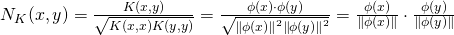where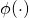is the implicit projection function operated by the kernel K. The normalization operation corresponds to a dot product in the RKHS of the normalized projections of the input instances. When K is LinearKernel on two vectors, the NormalizationKernel equals to the cosine similarity between the two vectors. The normalization operation is required when the instances to be compared are very different in size, in order to avoid that large instances (for instance long texts) are associated with larger similarities. For instance it is usually applied to tree kernels, in order properly compare trees having very different sizes.

Parameters:

• baseKernel: the base kernel whose output is enriched by this kernel

### References

John Shawe-Taylor and Nello Cristianini. Kernel Methods for Pattern Analysis. Cambridge University Press, New York, NY, USA, 2004. ISBN 0521813972.